Dave's Math Tables: Fourier Transforms(Math | Advanced | Transforms | Fourier)

### Fourier Transform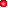Definition of Fourier Transform
f(x) = 1/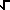(2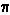)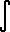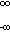g(t) e^(i tx) dtInverse Identity of Fourier Transform
g(x) = 1/(2)f(t) e^(-i tx) dt### Fourier Sine and Cosine TransformsDefinitions of the Transforms
f(x) =(2/)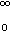g(x) cos(xt) dt (Cosine Transform)
f(x) =(2/)g(x) sin(xt) dt (Sine Transform)Identities of the Transforms
IF f(x) is even, THEN FourierSineTransform( FourierSineTransform(f(x)) ) = f(x)
IF f(x) is odd, THEN FourierCosineTransform( FourierCosineTransform(f(x)) ) = f(x)
Under certain restrictions of continuity.